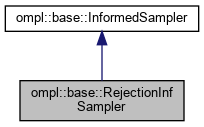ompl::base::RejectionInfSampler Class Reference

A default rejection sampling scheme that samples uniformly from the entire planning domain. Samples are rejected until one is found that has a heuristic solution estimate that is less than the current solution. In general, direct sampling of the informed subset is much better, but this is a general default. More...

#include <ompl/base/samplers/informed/RejectionInfSampler.h>

Inheritance diagram for ompl::base::RejectionInfSampler:[legend]

## Public Member Functions

RejectionInfSampler (const ProblemDefinitionPtr &probDefn, unsigned int maxNumberCalls)
Construct a rejection sampler that only generates states with a heuristic solution estimate that is less than the cost of the current solution.

bool sampleUniform (State *statePtr, const Cost &maxCost) override
Sample uniformly in the subset of the state space whose heuristic solution estimates are less than the provided cost, i.e. in the interval [0, maxCost). Returns false if such a state was not found in the specified number of iterations.

bool sampleUniform (State *statePtr, const Cost &minCost, const Cost &maxCost) override
Sample uniformly in the subset of the state space whose heuristic solution estimates are between the provided costs, [minCost, maxCost). Returns false if such a state was not found in the specified number of iterations.

bool hasInformedMeasure () const override
Whether the sampler can provide a measure of the informed subset.

double getInformedMeasure (const Cost &) const override
The measure of the subset of the state space defined by the current solution cost that is being searched. As rejection sampling has no closed-form knowledge of the informed subset, the measure of the informed space is always the measure of the entire space.

double getInformedMeasure (const Cost &, const Cost &) const override
The measure of the subset of the state space defined by the current solution cost that is being searched. As rejection sampling has no closed-form knowledge of the informed subset, the measure of the informed space is always the measure of the entire space.Public Member Functions inherited from ompl::base::InformedSampler
InformedSampler (const InformedSampler &)=delete

InformedSampleroperator= (const InformedSampler &)=delete

InformedSampler (const ProblemDefinitionPtr &probDefn, unsigned int maxNumberCalls)
Construct a sampler that only generates states with a heuristic solution estimate that is less than the cost of the current solution. Requires a function pointer to a method to query the cost of the current solution. If iteration is required, only maxNumberCalls are attempted, to assure that the function returns.

virtual Cost heuristicSolnCost (const State *statePtr) const
A helper function to calculate the heuristic estimate of the solution cost for a given state using the optimization objective stored in the problem definition. More...

ProblemDefinitionPtr getProblemDefn () const

unsigned int getMaxNumberOfIters () constProtected Attributes inherited from ompl::base::InformedSampler
ProblemDefinitionPtr probDefn_
A copy of the problem definition.

OptimizationObjectivePtr opt_
A copy of the optimization objective.

StateSpacePtr space_
A copy of the state space.

unsigned int numIters_
The number of iterations I'm allowed to attempt.

## Detailed Description

A default rejection sampling scheme that samples uniformly from the entire planning domain. Samples are rejected until one is found that has a heuristic solution estimate that is less than the current solution. In general, direct sampling of the informed subset is much better, but this is a general default.

Definition at line 53 of file RejectionInfSampler.h.

The documentation for this class was generated from the following files: